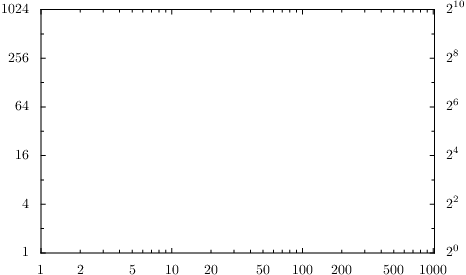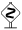# PyX — Example: axis/log.py

0.6 KB
62.5 KB
40.4 KB
18.8 KB
47.5 KB

## Logarithmic axes```import fractions, math
from pyx import *
from pyx.graph import axis

# we here use parters and texters which are explained in the examples below
log2parter = axis.parter.log([axis.parter.preexp([axis.tick.rational(1)], 4),
axis.parter.preexp([axis.tick.rational(1)], 2)])
log2texter = axis.texter.default(base=fractions.Fraction(2))

g = graph.graphxy(width=10,
x=axis.log(min=1, max=1024),
y=axis.log(min=1, max=1024, parter=log2parter),
y2=axis.log(min=1, max=1024, parter=log2parter, texter=log2texter))

g.writeEPSfile("log")
g.writePDFfile("log")
g.writeSVGfile("log")
```

### Description

Logarithmic axes are quite similar to linear axes and differ only in a very few properties, namely a non-linear mapping of values to axis positions. They also have different default partitioners and raters (see below). While the partitioner really is quite different - it implements a logarithmic positioning of the ticks - the rater just alters some defaults in favour of using more subticks to improve the visualization of the logarithmic nature of the axis.Besides presenting a standard logarithmic axis, we also show you how to alter the base used by the logarithmic axis. Since the functionality of a logarithmic axis is independent of the base, all we need to adjust are the components for creating ticks (see `log2parter`) and the texts for the labels (see `log2texter`). More details about the parters and texters components are given below in the corresponding examples.